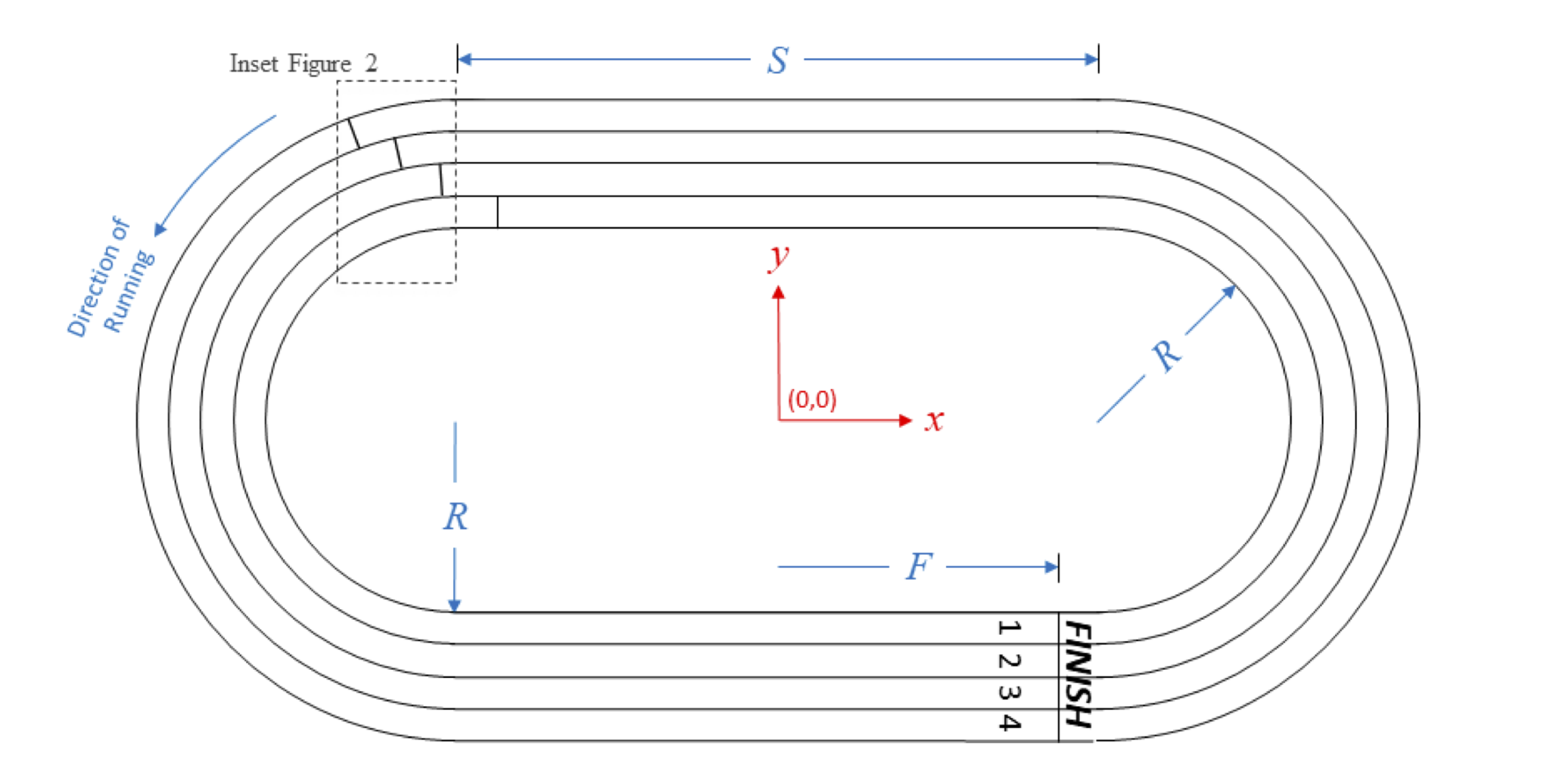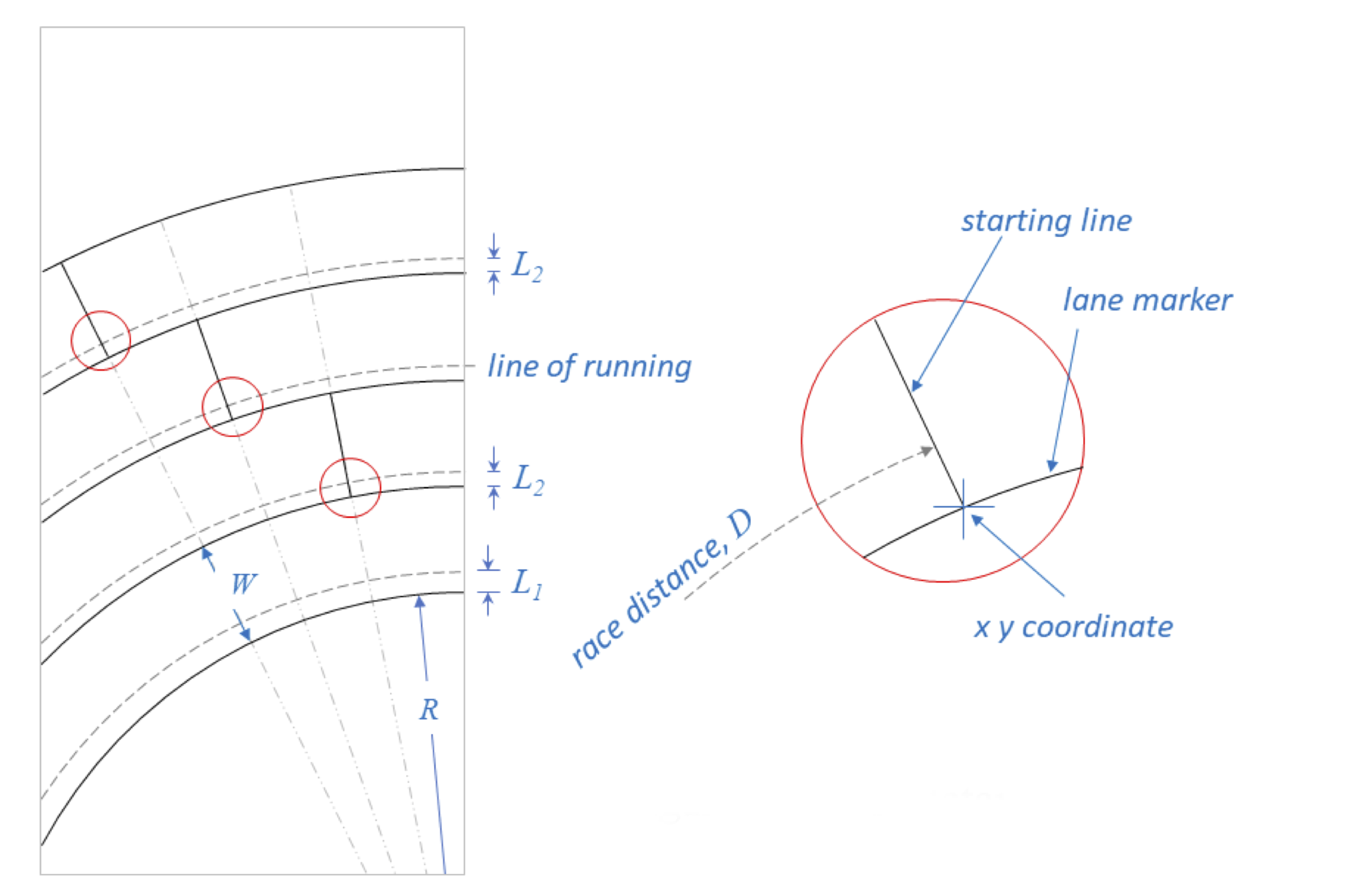시간 제한 메모리 제한 제출 정답 맞은 사람 정답 비율
1 초 1024 MB 1 1 1 100.000%

## 문제

An oval track and field racing track consists of two parallel straightaway sections connected by two semicircles, depicted in Figure 1.  Footraces run in the counterclockwise direction, ending at a common finish line located along the lower straightaway.  For races that exceed the length of a single straightaway, starting lines must be staggered backwards, in the clockwise direction, from the finish line.  The staggered starting lines must account for the curve of the semicircles and the widths of each running lane.Figure 1: Oval track with $200$m starting lines.Figure 2: Inset showing staggered starting line locations.

There are international standards for oval track dimensions. Unfortunately, the available area for a track doesn't always hold a standard track.  Given the dimensions of the track and the length of the race, your team is to write a program to ensure equal race lengths by computing the staggered starting line positions.

The total distance of a race for any given lane is computed from the line of running.  The line of running is an unmarked line to the right of the lane's inside marker (as seen from the counterclockwise direction).  See Figure 2.  For the innermost lane (lane 1) the line of running is usually farther from the lane marker than for the remaining lanes.

The track is mapped to an $(x, y)$ coordinate system with $(0, 0)$ at the center of the track.  See Figure 1.

## 입력

The first line of input to your program contains seven values, $N$ $R$ $S$ $W$ $F$ $L_1$ $L_2$, separated by whitespace, describing the geometry of a track, where:

• $N$ is the integer number of lanes. ($1 \le N \le 9$)
• $R$ is the inner radius of lane 1, a real number in meters.  See Figure 1.  ($1.0 \le R \le 100.0$)
• $S$ is the length of the straightaway, a real number in meters. See Figure 1.  ($1.0 \le S \le 200.0$)
• $W$ is the width of each lane, a real number in meters. See Figure 2.  ($0.5 \le W \le 3.0$)
• $F$ is the $x$-coordinate of the finish line, measured from the centerline in Figure 1, a real value in meters.  The finish line will always be in the lower (negative $y$) half of the track.  ($\vert F\vert \le S / 2$)
• $L_1$ is the offset from the inner radius of lane 1 to the line of running for lane 1, a real number in meters. See Figure 2.  ($0 \le L_1 < W$)
• $L_2$ is the offset from the inner radius to the lines of running for lanes 2 and higher.  See Figure 2.  ($0 \le L_2 < W$)

The remaining lines until end-of-file specify $D$, the distance of a race, one race per line, a real number in meters. ($1.0 \le D < 410.0$.) There will be at most $100$ distances $D$ in input.

## 출력

Your program is to print a series of values for each race distance, separated from each other by spaces and/or newlines. Print the race distance first, followed by the $(x, y)$ coordinates of the staggered starting line locations in lane number order. Express all values in meters.  The $(x, y)$ coordinate is the innermost point of a lane, NOT the line of running. Treat each lane marker (straightaway or radius) as a zero-width line. International standards require that the values be within $0.001$ meters of the exact answer.

## 예제 입력 1

4 36.5 84.39 1.22 40.0 0.30 0.20
200.0
400


## 예제 출력 1

200.000 -40.0006 36.5000 -43.5119 37.6970 -47.3108 38.6025 -51.0664 39.1679
400.000 40.0012 -36.5000 46.9998 -37.4127 54.4292 -36.9682 61.4438 -35.2464


## 출처• 문제를 만든 사람: Ed Skochinski